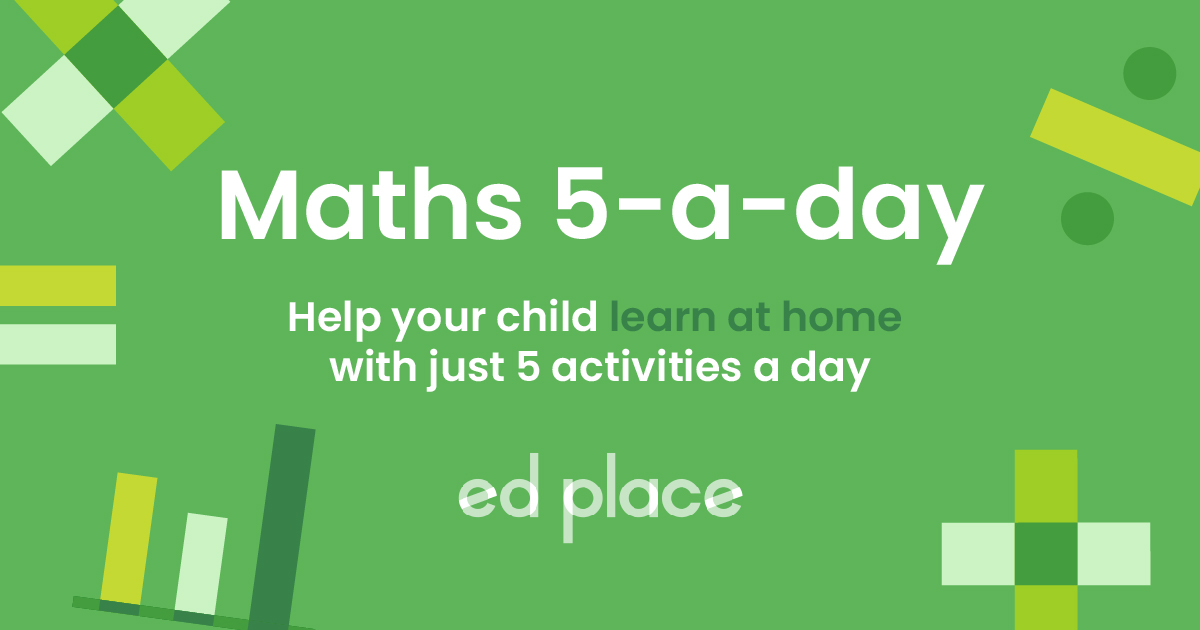# EdPlace's Year 6 Home Learning Maths Lesson: Order of Operations (BIDMAS)

Looking for short lessons to keep your child engaged and learning? Our experienced team of teachers have created English, maths and science lessons for the home, so your child can learn no matter where they are.  And, as all activities are self-marked, you really can encourage your child to be an independent learner.

Get them started on the lesson below and then jump into our teacher-created activities to practice what they've learnt. We've recommended five to ensure they feel secure in their knowledge - 5-a-day helps keeps the learning loss at bay (or so we think!).

Are they keen to start practising straight away? Head to the bottom of the page to find the activities.

Now...onto the lesson!

Key Stage 2 Statutory Requirements for Maths
Year 6 students should be able to use their knowledge of the order of operations to carry out calculations involving the four operations.

Think the answer to 6 + 4 x 5 = 50? Think again! There is a particular order in which we answer problems like this one and all your child needs to remember is BIDMAS. This article will help you teach your child the order of operations to correctly answer problems.

We're confident that if you follow this step-by-step approach together, your child will be able to:

1) Understand what BIDMAS is

2) Apply BIDMAS to independent work

3) Explain to you how they completed their work!

## Step 1 - Key terminology

Your child will need a basic understanding of the four operations: addition, subtraction, multiplication and division.

Indices (plural) – the index (singular) of a number shows how many times it is multiplied by itself.

For example, 52 = 5 x 5 = 25

53 = 5 x 5 x 5 = 125

## Step 2 -  Check your child's prior understanding

Your child will have learnt how to add, subtract, multiply and divide with increasingly larger numbers. When they get given questions involving the order of operations, the numbers are usually smaller. Fast recall of times tables and other number facts (such as number bonds) will be useful to your child.

## Step 3 - BIDMAS

The main thing you need to remember is: BIDMAS (or BODMAS)

Brackets

Indices

Division

Multiplication

Subtraction

In BODMAS, the O stands for Orders, which is the same as IndicesThis is the order in which to complete the four operations questions.

It’s actually shaped like a pyramid:Addition and subtraction have equal importance – so only those are done from left to right.

Division and multiplication can be done in any order – you will get the same answer either way:

5 x 4 ÷ 2 = 20 ÷ 2 = 10

5 x 4 ÷ 2 = 5 x 2 = 10

Examples:

1) 5 + 2 x 4 = 13

Multiplication comes before addition, so 2 x 4 = 8. Then we do 5 + 8 = 13.

2) 64 – 10 ÷ 2 = 59

Division comes before subtraction, so 10 ÷ 2 = 5. Then, 64 – 5 = 59.

3) 3 + (52 x 2) ÷ 10 = 8

25 x 2 = 50. Then 50 ÷ 10 = 5. Then 8 + 5 = 8

## Step 4 - Putting it into practice...

Now have a go at these examples together:

1) 6 + 4 x 5

2) 43 + 15 ÷ 3

3) 7 + 48 ÷ (18 – 16)

4) 22 – 4 + 10

Challenge questions:

Fill in the missing number

5) (26 – 10) ÷ _____ = 4

6) 4 x _____ - 25 = 23

Put the brackets in to make the answers correct

7) 8 x 9 – 5 – 6 = 26

## Step 5 - Give it a go...

Why not test your child's understanding and see if they can tackle these activities?

All activities are created by teachers and automatically marked. Plus, with an EdPlace subscription, we can automatically progress your child at a level that's right for them. Sending you progress reports along the way so you can track and measure progress, together - brilliant!

1) 26

4 x 5 = 20

6 + 20 = 26

2) 69

43 = 64

15 ÷ 3 = 5

64 + 5 = 69

3) 31

18 – 16 = 2

48 ÷ 2 = 24

7 + 24 = 31

4) 28

22 – 4 = 18

18 + 10 = 28 (addition and subtraction is left to right)

5) 4 (or 22)

6) 12

7) 8 x (9 – 5) – 6 = 26

Keep going! Looking for more activities, different subjects or year groups?

Click the button below to view the EdPlace English, maths, science and 11+ activity library